# Cartesian System

#### notes

On the number line, distances from a fixed point are marked in equal units positively in one direction and negatively in the other. The point from which the distances are marked is called the origin.
The number line to represent the numbers by marking points on a line at equal distances. If one unit distance represents the number ‘1’, then 3 units distance represents the number ‘3’, ‘0’ being at the origin. The point in the positive direction at a distance r from the origin represents the number r  where as the negative direction at a distance r from the origin represents the number -r. Fig.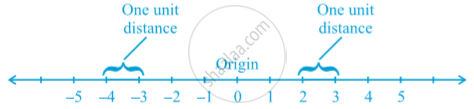The perpendicular lines  are actually obtained as follows : Take two number lines, calling them X′X and Y′Y. Place X′X horizontal as following  fig.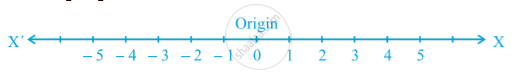and  the numbers on it just as written on the number line. We do the same thing with Y′Y except that Y′Y is vertical, not horizontal in following fig.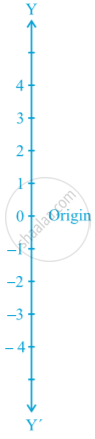Combine both the lines in such a way that the two lines cross each other at their zeroes, or origins in following fig.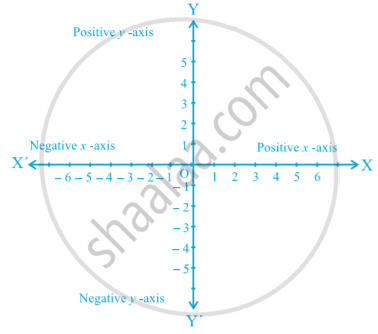The horizontal line X′X is called the x - axis and the vertical line YY′ is called the y - axis. The point where X′X and Y′Y cross is called the origin, and is denoted by O. Since the positive numbers lie on the directions OX and OY, OX and OY are called the positive directions of the x - axis and the y - axis, respectively. Similarly, OX′ and OY′ are called the negative directions of the x - axis and the y - axis, respectively.
The axes (plural of the word ‘axis’) divide the plane into four parts. These four parts are called the quadrants (one fourth part), numbered I, II, III and IV anticlockwise from OX in following fig.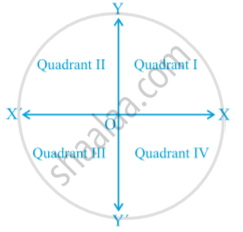The Cartesian plane, or the coordinate plane, or the xy-plane. The axes are called the coordinate axes.

If you would like to contribute notes or other learning material, please submit them using the button below.

### Shaalaa.com

Cartesian System [00:06:59]
S
0%